International
Tables for
Crystallography
Volume A
Space-group symmetry
Edited by M. I. Aroyo

International Tables for Crystallography (2015). Vol. A, ch. 1.5, p. 84

## Section 1.5.2.2. Metric tensors of direct and reciprocal lattices

H. Wondratscheka and M. I. Aroyob

#### 1.5.2.2. Metric tensors of direct and reciprocal lattices

| top | pdf |

The metric tensor of a crystal lattice with a basis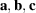is the (3 × 3) matrix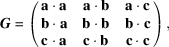which can formally be described as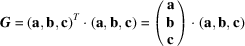(cf. Section 1.3.2). The transformation of the metric tensor under the coordinate transformationfollows directly from its definition: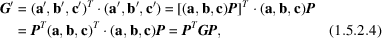where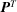is the transposed matrix of P. The transformation behaviour of G underis determined by the matrix P, i.e. G is not affected by an origin shift p.

The volume V of the unit cell defined by the basis vectorscan be obtained from the determinant of the metric tensor,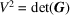. The transformation behaviour of V under a coordinate transformation follows from the transformation behaviour of the metric tensor [note that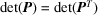]: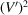=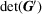=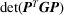=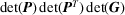=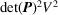, i.e.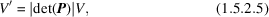which is reduced to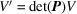if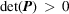.

Similarly, the metric tensorof the reciprocal lattice and the volumeof the unit cell defined by the basis vectorstransform as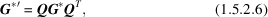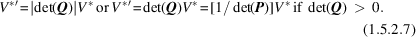Again, it is only the linear part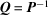that determines the transformation behaviour ofandunder coordinate transformations.